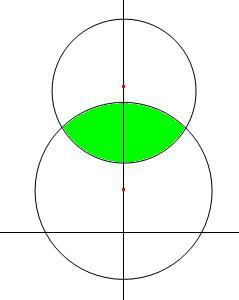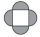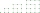# Four circles

1) Calculate the circle radius if its area is 400 cm square
2) Calculate the radius of the circle whose circumference is 400 cm.
3) Calculate circle circumference if its area is 400 cm square
4) Calculate the circle's area if perimeter 400 cm.

r1 =  11.2838 cm
r2 =  63.662 cm
o1 =  70.8982 cm
S2 =  12732.3954 cm2

### Step-by-step explanation:Did you find an error or inaccuracy? Feel free to write us. Thank you!#### You need to know the following knowledge to solve this word math problem:

We encourage you to watch this tutorial video on this math problem:

## Related math problems and questions:

• Area to perimeterCalculate circle circumference if its area is 254.34cm2
• Quarter of a circleCalculate the circumference of a quarter circle if its content is S = 314 cm2.
• RectangleThe length of the rectangle is 12 cm greater than 3 times its width. What dimensions and area this rectangle has if ts circumference is 104 cm.
• Two annulusesThe area of the annular circle formed by two circles with a common center is 100 cm2. The radius of the outer circle is equal to twice the radius of the inner circle. Determine the outside circle radius in centimeters.
• Perimeter of the circleCalculate the perimeter of the circle in dm, whose radius equals the side of the square containing 0.49 dm2?Calculate the radius of the circle whose length is 107 cm larger than its diameter
• SemicircleThe ornament consists of one square and four dark semicircles. The area of the square is 4 cm ^ 2. Find the area of one dark semicircle and round the result to hundreds.
• Circle - simpleCalculate the area of a circle in dm2 if its circumference is 31.4 cm.
• Two circlesTwo circles with a radius 4 cm and 3 cm have a center distance 0.5cm. How many common points have these circles?
• Trapezium basesFind the trapezium height if a = 8 cm and c = 4 cm if its content 21 square centimeters.The perimeter of a quadrilateral (four sided plane shape) is 125 cm . If the three known sides measure a=12 cm, b=45 cm, and c=30.5 cm, how long is the fourth side?The circular sector with a central angle 160° has an area 452 cm2. Calculate its radius r.How big is an area of a circle if its circumference is 51.2 cm?Question No. 1: The prism has the dimensions a = 2.5 cm, b = 100 mm, c = 12 cm. What is its volume? a) 3000 cm2 b) 300 cm2 c) 3000 cm3 d) 300 cm3 Question No.2: The prism base is a rhombus with a side length of 30 cm and a height of 27 cm. The height of tThe circumference and width of the rectangle are in a ratio of 5: 1. its area is 216cm2. What is its length?Find circumference and area of the rhumbline ABCD if the short side AD of which has a length of 5 cm, and the heel of the height from D leading to the AB side divides the AB side into two sections of 3 cm and 4 cm.Calculate the missing side of a parallelogram, if you know the perimeter o and one side: a) o = 7.2 cm; b = 1.8 cm b) o = 5.4 cm; a = 1.9 cm